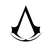# EOJ Monthly 2017.12 题解

ultmaster edited 2 年，1 月前

### A.

Prepared by kblack.

### B.

Prepared by kblack.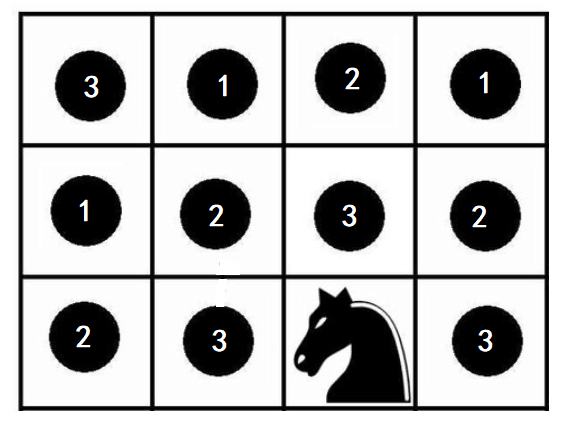### C.

Prepared by ultmaster.

### D.

Prepared by infiniteee.

### E.

Prepared by kblack.

Easy 部分可以简单的用 $dp[i][j][k]$: 买个 $i$ 根，最后一天买 $j$ 根，买 $j$ 根已经 $k$ 天来实现。朴素转移时间复杂度 $O(n^4)$ 足够通过，可是没人做。

$\prod_{i=x}^{n}(1+x^i+x^{2i}+\ldots+x^{(k-1)i})$
$= \prod_{i=x}^{n}\frac{1-x^{ki}}{1-x^i}$
$= \prod_{i=x}^{n}({1-x^{ki}}) \cdot \prod_{i=x}^{n}\frac{1}{1-x^i}$
$= \prod_{i=x}^{n}({1-x^{ki}}) \cdot \prod_{i=x}^{n}(1+x^i+x^{2i}+x^{3i}+\ldots)$

### F.

Prepared by ???.

Easy 可以简单地跑一遍 Floyd，然后发现是一个线性方程组，然后跑一下 Gauss Elimination。Done！

### G.

Prepared by BelowLuminous.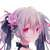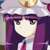C题再排回来是啥意思？？？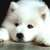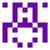F题每个点与其他点的差值ti怎么求啊@ultmaster E2 比昨天更多的棒棒糖 (Hard) 数组开不下怎么办